# Geometric Interpretation Of Schrodinger's

GEOMETRICAL STUDY OF SCHROEDINGER'S FORMULA

$$\vec J = \mathfrak{Re}[\Psi^* (\vec r) (\frac{-i \hbar}{m} \vec \nabla) \Psi (\vec r) ]$$

If we take a look on previous expression, we could continue with the importance of complex numbers.

The complex numbers are very important to represent points or vectors in plane, and can be expressed this way:

a = b·x+c·y

If we choose the real axis numbers like X, and imaginaries like Y:

a = b + c·i

But we could have been done this:

a = b·i + c

If we take Geometric Algebras (GA), more exactly the even sub group of GA, we can represent vectors like part quaternions, and rotations through quaternion operators.

If we take an element of volume (area in draw):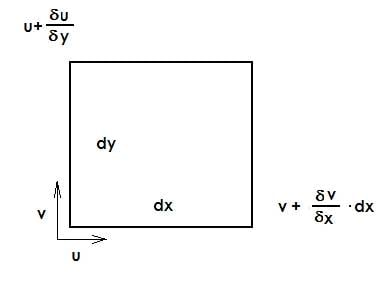And take a volume in a posterior time:

$$V_t+dt = \left( dx + \frac {\partial u}{\partial x} dx \cdot dt \right) \left( dy + \frac {\partial v}{\partial y} dy \cdot dt \right)$$

And the relative change of volume is:

$$\frac {d \left( \frac {V_t_+_d_t - V_t} {V_t} \right) } {dt} = \frac {dV_R}{dt} = \frac {\partial u}{\partial x} + \frac {\partial v}{\partial y}$$

We have that:

$$\frac {\partial V_R}{\partial t} = \frac {\partial u}{\partial x} + \frac {\partial v}{\partial y} + \frac {\partial w}{\partial z} = \nabla \cdot \vec {v}$$

The dilatation of volume can be directly expressed with velocity gradient in space:

A volume element can be:

TRANSLATED: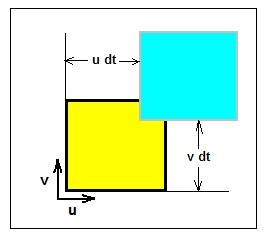DEFORMATED: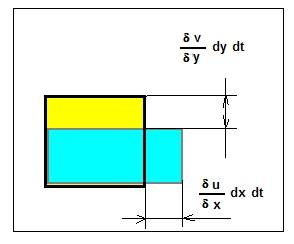ROTATED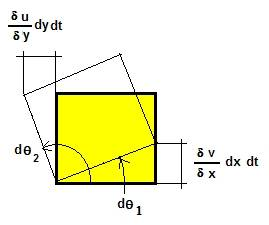or HAVE CUTTING EFFORTS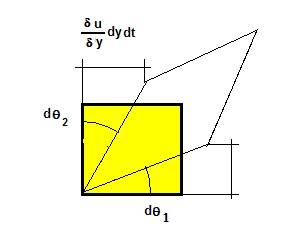If we analyze the angles $$\theta_1$$ y $$\theta_2$$, it can easily verify geometrically that:

$$TAN~~ d \theta_1 \simeq d \theta_1 \simeq \frac {\left( \frac {\partial v} {\partial x} dx \cdot dt \right) }{dx}$$

And:
$$TAN~~ d \theta_2 \simeq d \theta_2 \simeq \frac {\left( \frac {- \partial u} {\partial y} dy \cdot dt \right) }{dy}$$

And we have:

$$\dot {\theta_1} = \frac {d \theta_1}{dt} = \frac {\partial v}{\partial x}$$

and

$$\dot {\theta_2} = \frac {d \theta_2}{dt} = - \frac {\partial u}{\partial y}$$

And angular velocity in Z axis will be:

$$\omega_z = \frac {1}{2} \left( \frac {\partial v}{\partial x} - \frac {\partial u}{\partial y} \right) = \frac {1}{2} \dot {\theta_1} + \dot {\theta_2}$$

Now, if we take this fraction of the Schrödinger's:

$$\nabla \left[ \frac {i \hbar} {2m} \left( \psi \nabla \psi^*} - \psi^* \nabla \psi \right) \right]$$

Or much clear:

$$\nabla \left[ \frac {1} {2} \left( \psi \vec v \psi^*} - \psi^* \vec v \psi \right) \right]$$

Does anybody likes to play in discovering what it means?

-----------
References:

- Quaternion and Rotation Sequences, Jack B. Kuipers
- Vectors, tensors and the Basic Equations of Fluid Mechanics, Rutherford Aris.
- Vibraciones y ondas. A. P. French.
- Lectures On Clifford (Geometric) Algebras. Rafal Ablamowicz et. al.
- Mecánica de Fluidos. Victor L. Streeter et. al.
- De Natura Visibilium et Invisibilium. R. Aparicio. Ed. Elaleph.
- Siglo XXI: La Física que nos espera. R. Aparicio. Ed. Elaleph.[/quote]

Last edited:

Tom Mattson
Staff Emeritus
Gold Member
Plat00n,

I am having trouble figuring out what you are trying to do here, and it is mostly because I can't see the images you linked to. img tags don't work outside of the General Discussion Forum. If you want the images to be viewable here you'll need to attach them to a post.

Thanks,

Tom Mattson said:
Plat00n,
I am having trouble figuring out what you are trying to do here, and it is mostly because I can't see the images you linked to. img tags don't work outside of the General Discussion Forum. If you want the images to be viewable here you'll need to attach them to a post.
Thanks,

You can see it in the blogspot, that I have used to link it. Here is:

http://denaturavisibiliumetinvisibilium.blogspot.com/

Thanks.

Now images can be seen.

Anything to say?

nrqed
Homework Helper
Gold Member
Tom Mattson said:
Plat00n,

I am having trouble figuring out what you are trying to do here, and it is mostly because I can't see the images you linked to. img tags don't work outside of the General Discussion Forum. If you want the images to be viewable here you'll need to attach them to a post.

Thanks,

Hi Tom....

I saw the interesting thread on your class on differential forms (using Bachman`s book). I would like to discuss differential forms at a very elementary level... I hope you can help clarifying some points. I will post later this weekend in the diff geometry board. I hope you will be around to help me understand!

Regards,

Pat

jtbell
Mentor
Plott029 said:

Perhaps more people who might be interested in discussing this would have seen it if you had posted it in the Quantum Physics forum instead of here (one of the homework-help forums). I saw this thread for the first time today.

You could try posting a link to this thread in the Quantum Physics forum, or maybe the moderators would be willing to move this thread over there. I think they wouldn't like it if you re-post your entire first post there.